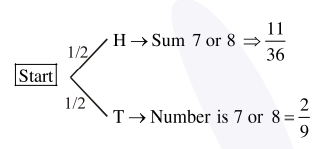# An unbiased coin is tossed. If the outcome is a head then a pair of unbiased dice is rolledQuestion:

An unbiased coin is tossed. If the outcome is a head then a pair of unbiased dice is rolled and the sum of the numbers obtained on them is noted. If the toss of the coin results in tail then a card from a well-shuffled pack of nine cards numbered $1,2,3, \ldots, 9$ is randomly picked and the number on the card is noted. The probability that the noted number is either 7 or 8 is :

1. $\frac{13}{36}$

2. $\frac{19}{36}$

3. $\frac{19}{72}$

4. $\frac{15}{72}$

Correct Option: , 3

Solution:$\mathrm{P}(\mathrm{A})=\frac{1}{2} \times \frac{11}{36}+\frac{1}{2} \times \frac{2}{9}=\frac{19}{72}$